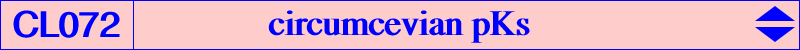Let pK(ω, π) be a pivotal cubic meeting the circumcircle (O) of ABC at A, B, C and three other points Q1, Q2, Q3. See the FG paper How pivotal cubics intersect the circumcircle. Q1Q2Q3 is the circumcevian triangle ccvP of some point P = p : q : r if and only if : • ω lies on the cubic Kω(P) with equation : ∑ q r (c^2 q + b^2 r) x^2 (c^4y - b^4z) + (b^2 p - a^2 q) (a^2 r - c^2 p) (c^2 q - b^2 r) x y z = 0 • π lies on the cubic Kπ(P) with equation : ∑ a^2 q r (c^2 q + b^2 r) x^2 (c^2y - b^2z) - (b^2 p - a^2 q) (a^2 r - c^2 p) (c^2 q - b^2 r) x y z = 0 Let S be the cevapoint of X(6), P and let T = P x S be the barycentric product of P, S. It is easily seen that Kω(P) = psK(X32 x T, T, P^2) and Kπ(P) = psK(T, X76 x T, P) which passes through Q1, Q2, Q3. Remark 1 : by adding the two equations above, we see that the two cubics Kω(P) and Kω(P) generate a pencil containing pK(X6 x T, X141 x T). This pK passes through its isopivot X(251) and the tangents at A, B, C pass through X(251). Remark 2 : the following assertions are equivalent : • P lies on the McCay cubic K003. • Kω(P) passes through X(6), hence there is an isogonal pK passing through Q1, Q2, Q3. • Kπ(P) passes through X(4), hence there is a pK with pivot X(4) passing through Q1, Q2, Q3. • there is a pK with isopivot X(3) passing through Q1, Q2, Q3. *** Since P lies on Kπ(P), it is easy to find infinitely many other points on the curve. Indeed, let F1(M) be the Ceva-conjugate of X76 x T and T ÷ M (barycentric quotient) and let F2(M) = T ÷ (Ceva-conjugate of X76 x T and M). F2 is the inverse of F1. Taking P = P(0), we define P(n - 1) = F1(P(n)) or P(n +1) = F2(P(n)). In this case, P(i), P(j), P(k) are collinear on Kπ(P) if and only if i + j + k = 0 and the tangential of P(i) is P(-2i). Each pivot P(n) on Kπ(P) corresponds to a pK denoted PK(n) with pole Ω(n) on Kω(P). PK(n) passes through Q1, Q2, Q3 and meets the lines AQ1, BQ2, CQ2 again at three collinear points S1, S2, S3 on a line L(n) passing through X(6). Two cubics PK(i) and PK(j) share the same points S1, S2, S3 if and only if i + j + 1 = 0. PK(0) being the union of the cevian lines of P, we see that PK(-1) is the only pK passing through P. About PK(-1) ... This is pK(X6 x S, agP x S) where agP is the anticomplement of the isogonal conjugate gP of P. When P = X(6), PK(-1) is the union of the cevial lines of X(6). This is excluded in the sequel. Recall that PK(-1) passes through P which is a point of inflexion with tangent passing through X(6). PK(-1) passes through gcgP and the vertices Pa, Pb, Pc of the circumcevian triangle of P. The tangents at these four points concur at X, the Ceva conjugate of the pivot and P. X is called TCC-perspector of P in ETC, see preamble just before X(1601). It contains gagP (its isopivot) hence the tangents at A, B, C and agP x S concur at gagP. A locus property : ccvP and acvM (anticevian triangle of M) are perspective if and only if M lies on pK(X6 x S, S), see CL043. The locus of the perspector is PK(-1). Note that pK(X6 x S, S) meets (O) at A, B, C and again at three points where the tangents are also concurrent at the Lemoine point of the triangle formed by these three points. The following table shows a selection of the most remarkable examples.P X(1) X(2) X(3) X(4) X(21) X(28) X(55) S X(81) X(83) X(2) X(275) X(14534) X(40395) X(1) X X(3) X(22) X(1498) X(24) X(1610) X(1612) X(1615) pK(X6 x S, S) K319 K644 K002 ? K379 K1174 pK(X31, X1) PK(-1) K318 K959 K004 K919 K1173 ? K1253Here is a list of pairs {P, X} taken from ETC. {1, 3}, {2, 22}, {3, 1498}, {4, 24}, {5, 1601}, {6, 6}, {7, 1602}, {8, 1603}, {9, 1604}, {13, 1605}, {14, 1606}, {15, 24303}, {17, 1607}, {18, 1608}, {19, 1609}, {21, 1610}, {25, 1611}, {28, 1612}, {31, 1613}, {32, 33786}, {35, 33669}, {54, 1614}, {55, 1615}, {56, 1616}, {57, 1617}, {58, 595}, {59, 1618}, {63, 1619}, {64, 1620}, {75, 33801}, {76, 33802}, {81, 1621}, {83, 1078}, {84, 1622}, {86, 23374}, {88, 1623}, {162, 1624}, {163, 1625}, {174, 1626}, {188, 2933}, {249, 33803}, {251, 1627}, {254, 1628}, {259, 198}, {266, 56}, {275, 1629}, {284, 1630}, {365, 55}, {366, 1631}, {508, 23852}, {509, 1486}, {512, 33704}, {523, 30715}, {648, 1632}, {651, 1633}, {662, 1634}, {1126, 33771}, {1171, 33774}, {2153, 11142}, {2154, 11141}, {3445, 33804}, {5374, 159}, {6727, 23846}, {6733, 23845}, {14085, 101}, {14086, 30715}, {14089, 99}, {14090, 33704}, {18297, 23849}, {18753, 2176}, {20034, 25}.